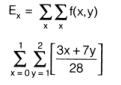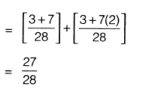Courses

# Electronic Devices & Digital Communications - MCQ Test - 2

## 20 Questions MCQ Test GATE ECE (Electronics) 2022 Mock Test Series | Electronic Devices & Digital Communications - MCQ Test - 2

Description
This mock test of Electronic Devices & Digital Communications - MCQ Test - 2 for Electronics and Communication Engineering (ECE) helps you for every Electronics and Communication Engineering (ECE) entrance exam. This contains 20 Multiple Choice Questions for Electronics and Communication Engineering (ECE) Electronic Devices & Digital Communications - MCQ Test - 2 (mcq) to study with solutions a complete question bank. The solved questions answers in this Electronic Devices & Digital Communications - MCQ Test - 2 quiz give you a good mix of easy questions and tough questions. Electronics and Communication Engineering (ECE) students definitely take this Electronic Devices & Digital Communications - MCQ Test - 2 exercise for a better result in the exam. You can find other Electronic Devices & Digital Communications - MCQ Test - 2 extra questions, long questions & short questions for Electronics and Communication Engineering (ECE) on EduRev as well by searching above.
QUESTION: 1

### A sample of germanium is doped to the extent of 1014 donor atoms/cm3 and 7 x 1013 acceptor atoms/cm3. The resistivity of pure germanium is 60 Ω cm. If the applied electric field is 2V/cm, then the total conduction current density is given by

Solution: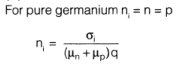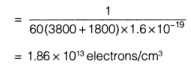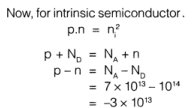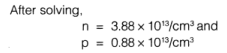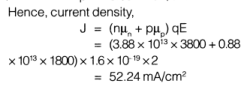QUESTION: 2

### An abrupt p-n junction diode is formed with two sides of resistivities 2 Ω cm in the p-side and 1 Ω cm on the n-side. The height of potential energy barrier for Ge (germanium) is

Solution: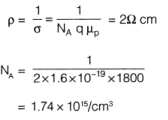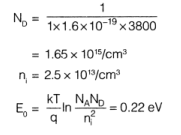QUESTION: 3

### An ideal p-channel MOSFET has the parameters. W = 15 μm, μp = 300 cm2A/ sec, L = 15 [μm, tox = 350 A° and VT = -0.80 V. If transistor is operating in non saturation region at VSD = 0.5 V, then the value of gm is ____________ μS

Solution: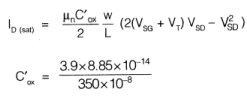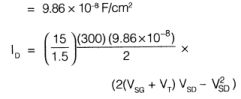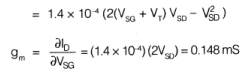QUESTION: 4

A Varactor diode has depletion capacitance given by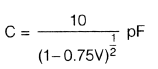where V is the bias voltage on the diode in volts. The diode is placed in parallel with a 0.75 (μH inductor which forms part of the frequency modulator. The required bias voltage so that the inductor diode combination resonates at 100 MHz is given by

Solution: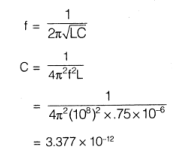QUESTION: 5

Figure shown is a battery charging circuit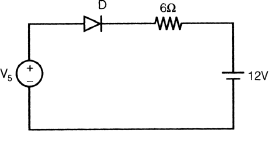If Vs = 120 sinωt, the conduction angle of the diode D is given by (i.e. in positive half cycle for how much total angle it conducts)

Solution: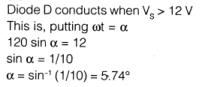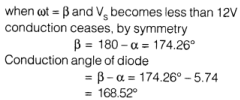QUESTION: 6

If the magnitude of the zener voltage is 4.9 V, what will be the current in the circuit? (The reverse saturation current is 5 μA).

Solution:
QUESTION: 7

In the BJT current source shown in figure, the diode voltage and the transistor base to emitter voltage are equal. If base current is neglected then collector current is __________ mA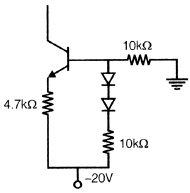Solution: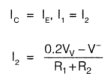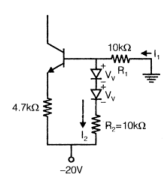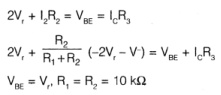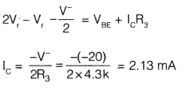QUESTION: 8

Two p-n germanium diodes are connected in series opposing. A 5V battery is impressed upon this series arrangement.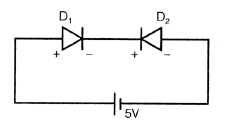The voltage across reverse bias p-n junction (Assume that the magnitude of the zener voltage is greater than 5V.

Solution: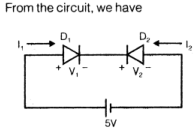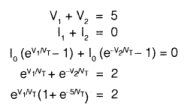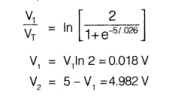QUESTION: 9

The parameters of an FET are gm = 3 mA/V, rd = 30 k, RL = 3k as an source follower load. The output impedance is given by

If R2 is replaced by a capacitor, then circuit behaves as

Solution: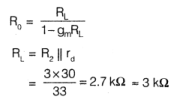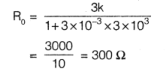QUESTION: 10

The N-channel FET having lDSS = 1 mA Vp = -1 V. The value of R1 when quiescent drain to ground voltage is 10 V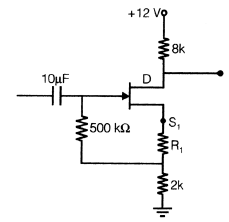Solution: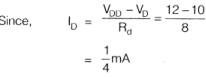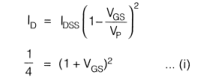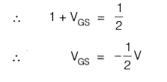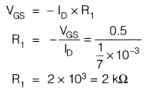QUESTION: 11

Diode current in circuit below for R = 20 kΩ is? When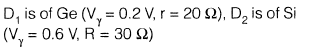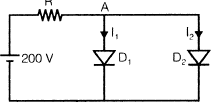Solution: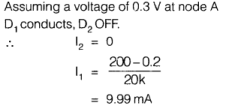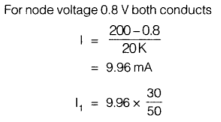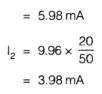QUESTION: 12

The ramp signal m(t) = at is applied to a delta modulator with sampling period “Ts” and step size “δ” then slope over load distortion would occur if,

Solution: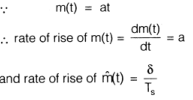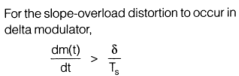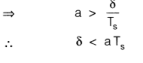QUESTION: 13

Consider the transistor circuit given below having an ideal diode: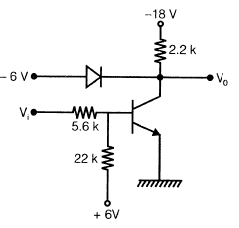Q.

The minimum value of hfe required neglecting saturation operation and assuming an ideal diode is

Solution: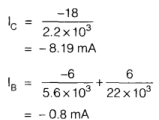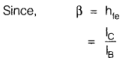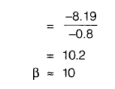QUESTION: 14

Consider the transistor circuit given below having an ideal diode:Q.

The maximum temperature at which the inverter would operate properly if the reverse saturation current at 25°C is 5 |xA is

Solution: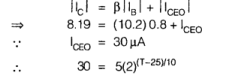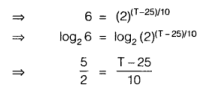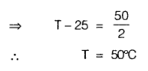QUESTION: 15

In a PCM system , if the quantization levels are increased from 2 to 16, the relative BW requirement will

Solution: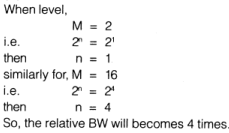QUESTION: 16

A random variable has following probability function.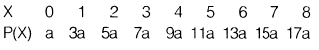Q.

Value of a is

Solution:
QUESTION: 17

A random variable has following probability function.Q.

Value of P (X < 4)

Solution:
QUESTION: 18

For a low pass filter circuit shown below if auto correlation function of input process x(t) is given by,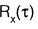= 5 δ(t) then,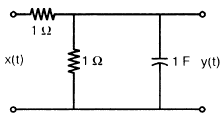Q.

PSD of the output random process is

Solution: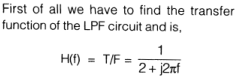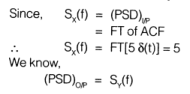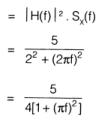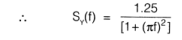QUESTION: 19

For a low pass filter circuit shown below if auto correlation function of input process x(t) is given by,= 5 δ(t) then,Q.

Average power of output random process is

Solution: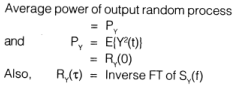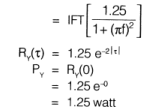QUESTION: 20

E(x) for joint probability function of 2 discrete random variables x and y given as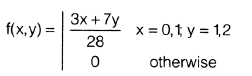Solution: